Next: The theory of collisions Up: Criticism of the conventional interpretation Previous: Spin and the Thomas precession   Contents

## Once again on mass

The mass conservation law, as an independent law, is confirmed by a vast amount of the experimental data. The elementary particles either do not change at all (but change their kinetic energy and the energy of their concordant electromagnetic field), or completely transform into the other particles. The photon is also a particle, which can be characterized by the velocity and frequency or by the wavelength. Simply, no arbitrary mass transformation into energy does exist.

Still remain in SRT the questions for particles with a zero rest mass. First, from relativistic expressions for energy and momentum in no way follows a rigorous transition to the case of. How, for example, can arise a continuum of every possible frequencies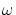in such a transition? Second, where do the gravitation energy (field) and the bending of space disappear (and where is their center of localization positioned at annihilation), if we have a linear chain of sequentially annihilating and born pairs, or in the case, where from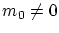we obtain, by means of reflections,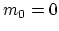? Generally speaking, the problem of photon's rest mass is senseless in the modern interpretation. The photon, as a definite particle, is characterized by some definite frequency. At rest (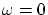) the photon would even be not a different particle; simply, it would cease to exist. Therefore, there is no concept of photon's rest mass (as well as the concept of photon's rest energy, etc.). On the other hand, for a real photon it is quite possible to determine not only the energy and momentum, but the mass as well. In the textbook  the conclusion was drawn quite incorrectly, that the particles with zero rest mass can not exist in the classical physics, since for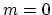any force must allegedly cause infinite acceleration. First, not any force can act on a photon with. For example, when the gravitation force acts, a zero mass is correctly "canceled" and the acceleration remains finite. Second, both the classical mechanics and SRT do not impose principal limitations on the value of acceleration. This allows one, for example, to consider the collisions of particles and the reflection of light to be instantaneous processes. Third, why the SRT choice is better, when under an effect of force, according to relativists' logic, the acceleration for light remains to be zero? If we appeal to intuition, then the infinite photon mass is obtained in SRT.

The field (possibly, not only electromagnetic?), as a material medium capable of transferring energy and possessing a momentum, can possess a mass as well (such a concept is inner consistent, but an experiment only can give an answer - whether this possibility is realized or not). Hence, for the classical physics it is also not surprising at all, that some field is capable to transfer the mass. In such a case the field must participate in the classical mass conservation law, and then the mass will be conserved in any reactions. The field must also participate in the momentum and energy conservation laws, and then one can not change the classical part of these conservation laws, which relates to particles. Therefore, in the classical physics it is also not surprising at all, that the excited atom can weigh greater than unexcited one, or the body with a greater energy can possess greater mass (by the way, it is impossible to verify this fact with the modern measurement precision). This additional mass is concentrated in the field, which causes particles to oscillate, to move over forceless trajectories or to kick from a particle-retaining wall. If we suppose the particles and the process of their collision itself to possess a purely electromagnetic nature, then in vacuum it is possible to use the relativistic expressions for the momentum-energy, but only from the viewpoint of unambiguous interrelations between quantities. However, one should remember here, that in this case the energy and momentum characterize the given collision process only, because they are written down, actually, with implicit allowance for the energy and momentum of the field (without explicit accounting and separating).Next: The theory of collisions Up: Criticism of the conventional interpretation Previous: Spin and the Thomas precession   Contents
Sergey N. Arteha